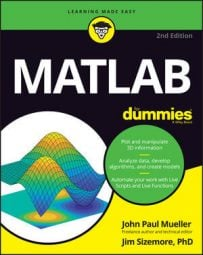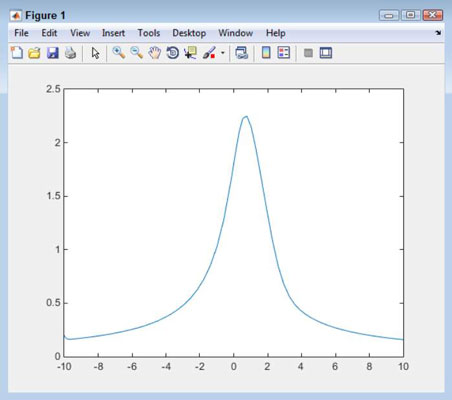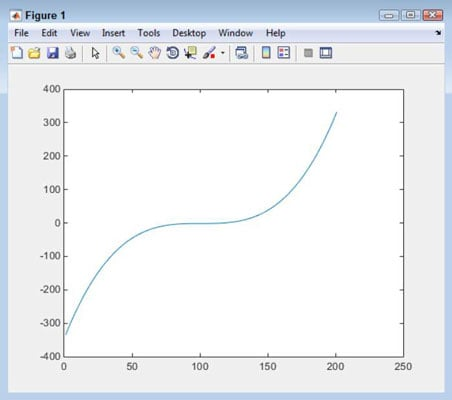##### MATLAB For DummiesWhen working with differential equations, MATLAB provides two different approaches: numerical and symbolic. Here, you can see both approaches to solving differential equations. This is just an overview of the techniques; MATLAB provides a rich set of functions to work with differential equations.

## Using the numerical approach

When working with differential equations, you must create a function that defines the differential equation. This function is passed to MATLAB as part of the process of obtaining the result.

There are a number of functions you can use to perform this task; each has a different method of creating the output. Check out a list of these functions. This example uses ode23(), but the technique works for the other functions as well.

MATLAB has a specific way of looking at your function. The order in which the variables appear is essential, so you must make sure that your function is created with this need in mind. This example simplifies things to avoid the complexity of many examples online and let you see the process used to perform the calculation. The following steps get you started:

1. Type Func = @(T, Y) cos(T*Y) and press Enter.

You see an output of

```Func =
@(T,Y)cos(T*Y)```

Many of the sources you see will tell you that you must place the equation in a separate function file on disk. However, this example demonstrates that creating a temporary function works just fine.

The requirements for the differential function are that you must provide an input for time and another input containing the values for your equation. The time value, T, is often unused, but you can use it if you want. The variables can consist of anything required to obtain the result you want. In this case, you input a simple numeric value, Y.

2. Type [TPrime, YPrime] = ode23(Func, [-10, 10], .2); and press Enter.

When using ode23(), you must provide a function — Func in this case — as input. As an alternative, you provide the name of the file containing the function. The second argument is a vector that contains the starting and ending times of the calculation. The third argument is the starting input value for the calculation.

The TPrime output is always a vector that contains the time periods used for the calculation. The YPrime output is a vector or matrix that contains the output value or values for each time period. In this case, YPrime is a vector because there is only one output value.

3. Type plot(TPrime, YPrime) and press Enter.

You see the plotted result for this example.## Using the symbolic approach

When working with the symbolic approach, you rely on the functionality of the Symbolic Math Toolbox to speed the solution along and make it a little easier to solve. The symbolic approach is a little more straightforward than the numerical approach. When using the symbolic approach, you rely on dsolve(). The following steps show a simple example of using dsolve() to create a differential solution and then plot it:

1. Type Solution = dsolve(‘Dy=(t^2*y)/y', ‘y(2)=1', ‘t') and press Enter.

The arguments to dsolve() consist of the equation you want to solve, the starting point for y (a condition), and the name of the independent variable. You see the following output from this entry:

```Solution =
t^3/3 - 5/3```
2. Type Values = subs(Solution, ‘t', -10:.1:10); and press Enter.

Solution simply contains the solution to the equation given the conditions you provide. The subs() function substitutes values for t one at a time. In this case, the values range from –10 to 10 in 0.1 increments. When this command completes, Values contains a list of results for the values you provided that you can use as plot points.

3. Type plot(Values) and press Enter.